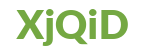# Data Science using Python Training

Data Science using PythonTotal Learners

0 LearnersCourse Duration

0 Hours

## Data Science using Python Training Course Syllabus

• In this module, participants will be introduced to Descriptive and Inferential statistics concepts and implementation using real-world data will also be covered. It will end with a case study implementation of EDA.

• Statistics Fundamentals and Implementation
• Exploratory Data Analysis
• ESSENTIALS OF PYTHON PROGRAMMING
• BASIC DATA STRUCTURES AND FUNCTIONS IN PYTHON
• INTRODUCTION TO
-Numpy Library
-Pandas Library
-Matplotlib and Seaborn Library
• DATA EXPLORATION USING STATISTICS
• Machine Learning algorithms are the backbone of Predictive Modelling. This is where the Crux of Data Science lies. The end objective of solving a data science problem is finding the patterns in the data and represent that in the form of a Data model. The algorithms taught in our course cover almost all of the problems data scientists solve on a regular basis.

• Introduction to Supervised and Unsupervised Learning
• Linear Regression with Multiple Variables
• Logistic Regression
• Decision Trees [CART]
• k-Fold Cross Validation
• Bagging and Bootstrapping
• Random Forest
• Principal component Analysis
• K-means clustering
• Hierarchical Clustering
• KNN
• Support Vector Machine
• Naive Bayes
• Time Series Analysis
• Tableau is one of the most popular Data Visualization tools used by Data Science and Business Intelligence professionals. In fact, it has been the market leader in reporting tools for almost 10 years (Source: Gartner magic quadrant). Once the predictive analysis of data is done, data scientists generally use Tableau to send out the reports to business which can then take decisions accordingly.

• INTRO TO DATA VISUALIZATION AND TABLEAU
• DATA CONNECTIONS – JOINS AND VIZQL
• BUIDING BASIC CHARTS, CHART TYPES AND MAPPING
• AGGREGATION, PARAMETER
• STATISTICAL ANALYSIS USING TABLEAU – REGRESSION AND BOX PLOTS
• TABLE CALCULATION, CALCULATED FIELDS
• DASHBOARDING
• INTEGRATION OF PYTHON WITH TABLEAU

## Data Science using Python Training Ratings

### 0

#### 0

H2K Infosys - IT Training & Placement Assistance
Rated 4.8/5 based on 198 customer reviews

### Request a DemoI agree to the H2KInfosys T&C